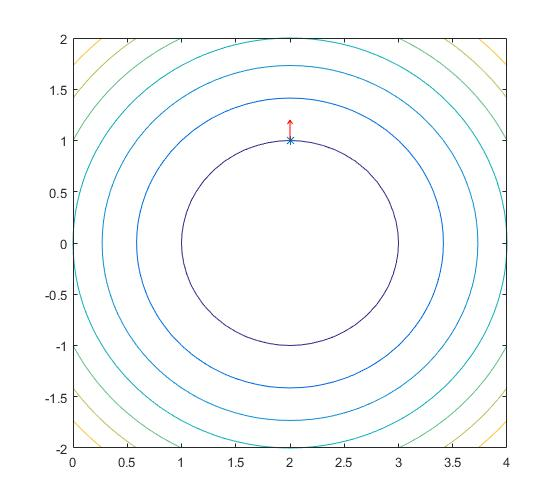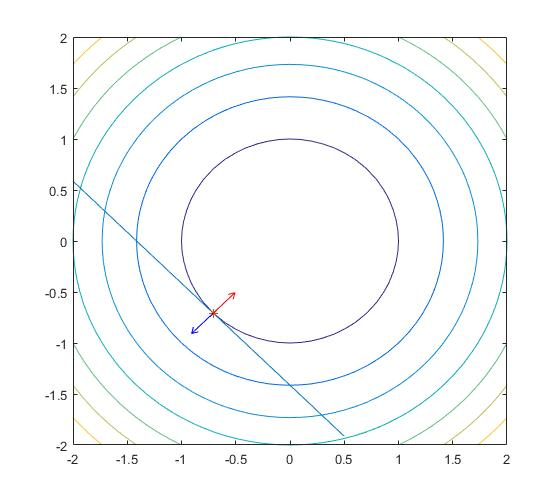## 拉格朗日函数及KKT条件

### 一个等式约束

1. 点$x$在超平面$h(x)=0$上的梯度方向垂直于超平面方向
2. 如果$f(x)$的最优值在$x^*$处取得，则$f(x)$在该点的梯度必垂直与超平面$h(x)=0$$f(x)$在直线与圆相切时的切点处取得最小值，这是直线的梯度方向如红色箭头所示，圆的梯度如蓝色箭头所示。

### 多个等式约束

(8)和(5)虽然看起来不一样，但同样可以用解释(5)的方式来解释(8)。实际上，每个等式确定的超平面梯度方向的加和必定与多个等式确定的超平面垂直。我们可以举个简单例子来验证这一点，比如我们有两个等式，两个等式确定的平面都是一个二维面，那么这两个等式联合确定的超平面就是条直线，由高中知识（不知道的去翻书）可知，这条直线垂直于这两个平面的法向量所确定的平面。

### 不等式约束

1. $x^{\ast}$出现在体中
2. $x^{\ast}$出现在体表面上

## 拉格朗日对偶

### Slater约束准则

Slater准则：当存在一个相对内点使得所有$g_i(x) \lt 0$严格成立时，强对偶性成立。如果$g_i(x)$中是仿射函数（$f(x)=c^{\mathrm T}x+t$这种函数），则不需要限制。